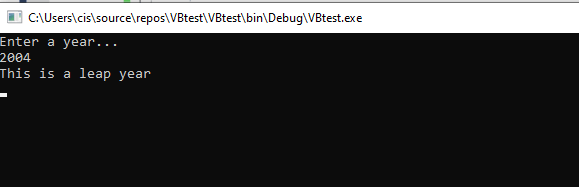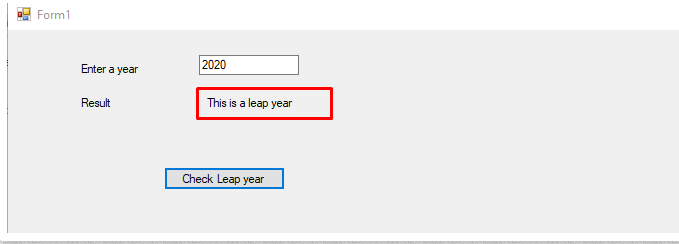# Visual basic program to check leap year or not

What is a leap year and how to write a visual basic program to check leap year? before write the code let’s understand the leap year.

# What is a leap year?

A year that has 366 days is called a leap year. It means an extra day in the year which will be 29 Feb and it comes in every 4 years.

As a programming logic, we can say A year which is divide by 4, 1oo and 400 is called a leap year.

## Visual basic program to check leap year or not

In the below code will solve the leap year problem using visual basic. It will take a year as user input and check the year is divisible 4, 100 and 400. If it is divisible then it will print “This is a leap year” or If it is not then it will pint “This is not a leap year”.

```Module Module1
Sub Main()
Console.WriteLine("Enter a year...")
Dim x, y, z, year
x = year Mod 4
y = year Mod 100
z = year Mod 400
If ((x = 0 And Not (y = 0)) Or z = 0) Then
Console.WriteLine("This is a leap year")
Else
Console.WriteLine("This is not a leap year")

End If
End Sub

End Module
```

Output:## Check leap year in visual basic using forms

This is the example 2 that is using Visual basic form or you can say a visual basic application. that going to implement the same logic but this time using forms.

It will take a year as input check the condition and print output message as a result.

```Public Class Form1
Dim x, y, z, year
Private Sub Button1_Click(sender As Object, e As EventArgs) Handles Sum.Click
year = TextBox1.Text
x = year Mod 4
y = year Mod 100
z = year Mod 400
If ((x = 0 And Not (y = 0)) Or z = 0) Then
Label2.Text = "This is a leap year"
Else
Label2.Text = "This is not a leap year"
End If
End Sub

End Class
```

Output### 2 thoughts on “Visual basic program to check leap year or not”

1.Mustapha

i dont undastooth well

2.mayur

Private Sub CMDleapyearcheck_click()

Dim X, year as Integer

year = Inputbox(“Enter Year”)
x = year mod 4

if X =0 then msgbox(“Leap Year”)
Else msgbox(“not Leap Year”)

End if
End sub